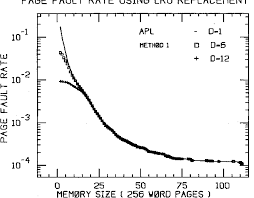## How to Calculate and Solve for Rate | Salt Trace Method | Water BudgetThe image above represents rate.

To compute for rate, three essential parameters are needed and these parameters are Rate of injection for salt solution (q), Concentration of salt solution (c1) and Concentration of river downstream (c2).

The formula for calculating rate:

Q = q(c1 – c2)

Where:

Q = Rate
q = Rate of Injection for Salt Solution or Trace
c1 = Concentration of Salt Solution or Trace
c2 = Concentration of River Downstream

Let’s solve an example;
Find the rate when the rate of injection for salt solution is 12, the concentration of salt solution is 20 and the concentration of river downstream is 18.

This implies that;

q = Rate of Injection for Salt Solution or Trace = 12
c1 = Concentration of Salt Solution or Trace = 20
c2 = Concentration of River Downstream = 18

Q = q(c1 – c2)
Q = 12(20 – 18)
Q = 12(2)
Q = 24

Therefore, the rate is 24.

Calculating the Rate of Injection for Salt Solution when the Rate, the Concentration of Salt Solution and the Concentration of River Downstream is Given.

q = Q / (c1 – c2)

Where;

q = Rate of Injection for Salt Solution or Trace
Q = Rate
c1 = Concentration of Salt Solution or Trace
c2 = Concentration of River Downstream

Let’s solve an example;
Find the rate of injection for salt solution when the rate is 40, the concentration of salt solution is 32 and the concentration of river downstream is 14.

This implies that;

Q = Rate = 40
c1 = Concentration of Salt Solution or Trace = 32
c2 = Concentration of River Downstream = 14

q = Q / (c1 – c2)
q = 40 / (32 – 14)
q = 40 / 18
q = 2.2

Therefore, the rate of injection for salt solution is 2.2.